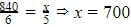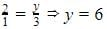# Test: Direct And Inverse Proportions- 2

## 20 Questions MCQ Test NCERT Mathematics for CAT Preparation | Test: Direct And Inverse Proportions- 2

Description
Attempt Test: Direct And Inverse Proportions- 2 | 20 questions in 20 minutes | Mock test for Class 8 preparation | Free important questions MCQ to study NCERT Mathematics for CAT Preparation for Class 8 Exam | Download free PDF with solutions
QUESTION: 1

### When one quantity is increased, the other quantity is also increased. This proportion is called .....................

Solution: It is called Direct proportion as it's one quantity is increased other also increases.
QUESTION: 2

### If y = x, then both are

Solution:

Since y=x, if y increases , x also increases and if y decreases x also decreases. So they are directly related.

QUESTION: 3

### 72 books are packed in 4 cartons of the same size. How many cartons are required for 360 books?

Solution: Correct answer is 'C' because in each cartoon there are 18 books. If we divide 360 /18 than the answer is 20.
QUESTION: 4

36 men can complete a piece of work in 18 days. In how many days will 27 men complete the same work ?

Solution: 36 men can complete a piece of work in 18 days. 1 Man can complete a piece of work in 36*18 27 men can complete a piece of work =36*18/27 =36*2/3 =12*2=24
QUESTION: 5

A garrison of 500 persons had provisions for 27 days. After 3 days a reinforcement of 300 persons arrived. For how many more days will the remaining food last now?

Solution:

Let the remaining food last for x days
500 men had provision for = (27 - 3) = 24 days
(500 + 300) men had provision for x days
More men, Less days (Indirect proportion)
∴ 800 : 500 :: 24 : x
⇔ (800 × x) = (500 × 24)
⇔ x = (500 × 24)/800
⇔ x = 15

QUESTION: 6

12 men can dig 8 meters long trench in a day. How many men should be employed for digging 50 meter long trench of the same type in one day?

Solution:
QUESTION: 7

In an army camp, there are 320 soldiers. They have sufficient food for 80 days. If after 20 days, 20 soldiers left the camp, for how many more days will the food last?

Solution:

Food for 320 students= 80 days

20 students left the campus

Number of students = 320 - 20 = 300 students

Food will last for (80 - 20) = 60 days fpr 320 students

Food will last for X days for 300 students

⇒ X = (320 x 60)/300 = 64

Food for 300 students= 64 days

QUESTION: 8

A journey by bus takes 45 minutes at 40 km/hour. How fast must a car go to undertake the same journey in 25 minutes?

Solution:
QUESTION: 9

The cost of 5 metres of a particular quality of cloth is Rs 210. Find the cost of 2 metres of cloth of the same type.

Solution:
QUESTION: 10

A mixture of paint is prepared by mixing 1 part of red pigments with 8 parts of base. How many parts of base will be used in mixture by mixing 4 part of red pigment?

Solution:
QUESTION: 11

6 pipes are required to fill a tank in 1 hour 20 minutes. How long will it take if only 5 pipes of the same type are used?

Solution:
QUESTION: 12

Two quantities x and y are said to be in ___________ if they increase (decrease) together in such a manner that the ratio of their corresponding values remains constant.

Solution:
QUESTION: 13

Two quantities x and y are said to be in _________ if an increase in x causes a proportional decrease in y (and vice-versa) in such a manner that the product of their corresponding values remains constant.

Solution:
QUESTION: 14

An electric pole, 14 metres high, casts a shadow of 10 metres. Find the height of a tree that casts a shadow of 15 metres under similar conditions.

Solution:
QUESTION: 15

A machine in a soft drink factory fills 840 bottles in six hours. How many bottles will it fill in five hours?

Solution:

It’s direct proportionBottles

QUESTION: 16

Suppose 2 kg of sugar contains 9 × 106 crystals. How many sugar crystals are there in 1.2 kg of sugar?

Solution:
QUESTION: 17

Two persons could fit new windows in a house in 3 days. One of the persons fell ill before the work started. How long would the job take now?

Solution:

It is an inverse relationdays

QUESTION: 18

The cost of 5 metres of a particular quality of cloth is Rs 210. Find the cost of 4 metres of cloth of the same type.

Solution:

Given, price of 5m cloth = 210
price of 1m cloth = 210 / 5 = 42
price of 4m cloth = 4×42
= RS.168

QUESTION: 19

A mixture of paint is prepared by mixing 1 part of red pigments with 8 parts of base. How many parts of base will be used in mixture by mixing 7 part of red pigment?

Solution:

It is direct proportion,
So, 1/8 = 7/y
y = 8 * 7
y = 56 parts of base.

QUESTION: 20

There are 100 students in a hostel. Food provision for them is for 20 days. How long will these provisions last, if 25 more students join the group?

Solution:

Number of person = 100, Number of days = 20, = 100 * 20
Number of person = 125  ( 25 student join), let Number of days = x = 125 * X
So, 100 * 20 = 125 * X
or 2000 = 125x
or, 125x =  2000

∴ X = 2000/125 = 16
Therefore, that the last provisions will 16 dyas.Use Code STAYHOME200 and get INR 200 additional OFF Use Coupon Code